# JP2006054801A - Video information composition simultaneous encoding apparatus - Google Patents

## Info

Publication number
JP2006054801A
JP2006054801A JP2004236620A JP2004236620A JP2006054801A JP 2006054801 A JP2006054801 A JP 2006054801A JP 2004236620 A JP2004236620 A JP 2004236620A JP 2004236620 A JP2004236620 A JP 2004236620A JP 2006054801 A JP2006054801 A JP 2006054801A
Authority
JP
Japan
Prior art keywords
rate
video information
fnm
encoding
master
Prior art date
Legal status (The legal status is an assumption and is not a legal conclusion. Google has not performed a legal analysis and makes no representation as to the accuracy of the status listed.)
Granted
Application number
JP2004236620A
Other languages
Japanese (ja)
Other versions
JP4249672B2 (en
Inventor
Yasuyuki Nakajima
Hiromasa Yanagihara
Akio Yoneyama

Original Assignee
Kddi Corp
Ｋｄｄｉ株式会社
Priority date (The priority date is an assumption and is not a legal conclusion. Google has not performed a legal analysis and makes no representation as to the accuracy of the date listed.)
Filing date
Publication date
Application filed by Kddi Corp, Ｋｄｄｉ株式会社 filed Critical Kddi Corp
Priority to JP2004236620A priority Critical patent/JP4249672B2/en
Publication of JP2006054801A publication Critical patent/JP2006054801A/en
Application granted granted Critical
Publication of JP4249672B2 publication Critical patent/JP4249672B2/en
Application status is Expired - Fee Related legal-status Critical
Anticipated expiration legal-status Critical

• 239000000203 mixtures Substances 0 abstract title 2
• 239000000872 buffers Substances 0 abstract 1
• 239000000047 products Substances 0 abstract 1
• 230000001603 reducing Effects 0 abstract 1
• 230000001629 suppression Effects 0 abstract 1

## Images

•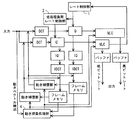•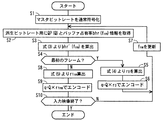•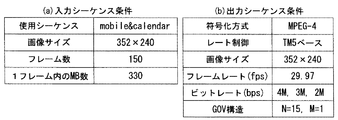•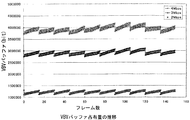•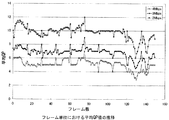•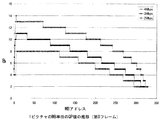•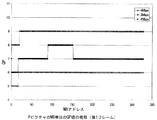•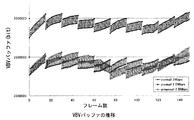•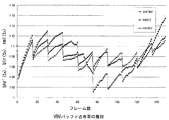•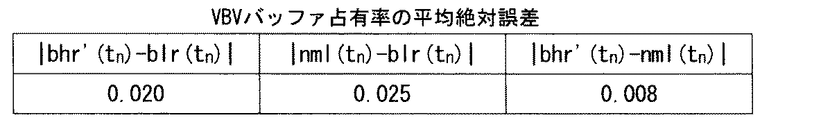•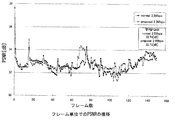•••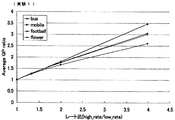•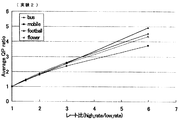•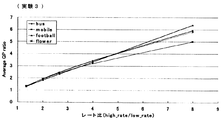••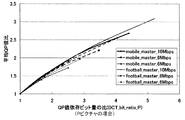•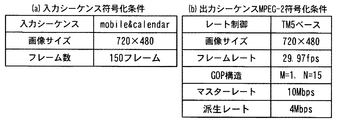••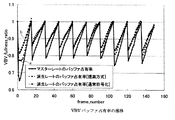•••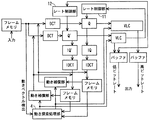## Abstract

<P>PROBLEM TO BE SOLVED: To provide a video information composition simultaneous encoding apparatus, capable of reducing the throughput of rate control, suppressing picture quality deterioration at a high speed, and performing encoding processing, even if the bit-rate ratio of the master rate and the derived rate is equal to 2 or larger. <P>SOLUTION: A rate control unit 1 for performing rate control of a quantizer Q of the master rate performs normal procedures, that is, processing of steps 1 to 3; whereas a rate control unit 2 for performing rate control of a quantizer Q' of the descendant rate uses a quantization parameter encoded at the master rate to encode a video. The quantization parameter of the descendant rate is obtained by the product of the quantization parameter Q at the encoding of the master rate and weighting coefficients (r), calculated on the basis of buffer occupancy. An initial value r0 of the weighting coefficients are obtained, only by the ratio of a bit quantity to be controlled by the quantization parameter (for example, the bit quantity of DCT coefficients). <P>COPYRIGHT: (C)2006,JPO&NCIPI

## Description

The present invention relates to a plurality of video information simultaneous encoding apparatuses, and more particularly to simultaneous encoding of a plurality of streams required for video distribution to various networks and playback environments at a higher speed than conventional methods and with reduced image quality degradation. The present invention relates to a plurality of video information simultaneous encoding devices that can be performed.

When generating video content, it is necessary to generate a plurality of contents having different bit rates according to various networks and playback environments. However, encoding video information individually takes a lot of time and effort. Accordingly, several transcoding schemes have been devised that simultaneously generate a plurality of streams at high speed.

An example of this will be briefly described with reference to the block diagram of FIG. The figure is a well-known MPEG2 multiple simultaneous encoding apparatus for video information, so detailed description of the operation will be omitted, and only the main points will be described. This conventional apparatus generates and outputs two types of streams of a low bit rate and a high bit rate, and each loop of low and high bit rates, that is, a DCT-quantization unit (Q) -inverse quantization unit (IQ) -inverse DCT (IDCT) -frame memory-motion compensation unit (MC), and two rate control units 11 and 12 for rate controlling the quantization units Q and Q ' ing. In this conventional apparatus, the processing is reduced by sharing the motion search processing unit 4.

As another conventional apparatus, for example, as described in “Research of MPEG2 transcoder quantization control system for realizing multi-rate output function” of Information Research Bulletin, AVM33-5 (2001). There is a method of partially sharing the processing.
"Study of MPEG2 transcoder quantization control method for realizing multi-rate output function" of Information Research Institute, AVM33-5 (2001)

The above-described conventional techniques reduce the amount of processing and increase the speed by using a method for sharing motion vector searches at low and high bit rates and a method for partially sharing quantization processing, respectively. The rate control processing required when encoding the stream of each bit rate is performed independently by the rate control units 11 and 12. No consideration has been given to reducing the amount of rate control processing.

The present invention has been made in view of the prior art, and an object of the present invention is to simultaneously encode a plurality of pieces of video information capable of performing encoding processing at a high speed while suppressing deterioration in image quality by reducing the amount of rate control processing. To provide an apparatus.

In order to achieve the above-described object, the present invention provides a video information multiple simultaneous encoding apparatus that simultaneously encodes a plurality of video information, a plurality of quantizers that perform quantization at a plurality of different bit rates, and the plurality of quantum information. A rate control unit for obtaining a quantization parameter of the encoder, the rate control unit encoding a video of a derived rate using the quantization parameter encoded at a master rate, and using the encoded rate video for the rate control The quantization parameter of the derived rate is obtained by the product of the quantization parameter Q at the time of master rate encoding and the weighting factor r calculated based on the buffer occupancy, and the initial value r 0 of the weighting factor r is The first feature is that it is obtained by the ratio of only the bit amount controlled by the quantization parameter.

Further, according to the present invention, the initial value r 0 is a value corresponding to a picture type, and the initial values r I0 and r P0 of the I picture and the P picture are obtained from the following equations (1) and (2), respectively. There is a second characteristic in the point to be obtained.
r I0 = A × (rate ratio of DCT coefficient of I picture) + B (1)
r P0 = C × (rate ratio of DCT coefficient of P picture) + D (2)
Here, A, B, C and D are constants.

According to the first to sixth aspects of the present invention, normal rate control is performed for the encoding of the master rate, and for the encoding at the derived rate, the encoding parameter obtained by the encoding of the master rate is performed. Therefore, the amount of processing required for rate control can be reduced, and the processing of a plurality of simultaneous encoding devices can be speeded up.

Even if the bit rate ratio is 2 or more, the initial value r 0 of the weighting coefficient r can be an appropriate value that does not depend on the input image conditions. In addition, the generated file capacity and the average PSNR are almost the same as those in the normal encoding, and even when the bit rate ratio to the master rate is 2 or more, the encoding of the derived rate is almost the same as the normal encoding. That is, it is possible to perform the image quality deterioration sufficiently.

Hereinafter, the present invention will be described in detail with reference to the drawings. First, normal rate control (MPEG-2 ISO / IEC13396-2 Test Model 5) processing will be described. In the normal rate control, the following steps 1 to 3 are performed.

(Step 1)
First, prior to encoding of each frame, the complexity indicators Xi, Xp, and Xb of I, P, and B pictures defined by the following equations are updated. Si, Sp, Sb is generated bit number, Qi (ave), Qp (ave), Qb (ave) is an average quantization parameter (hereinafter referred to as QP) (average value of mquant of all MBs in one frame, however Normalized to the range of 1 to 31). The complexity index is large for an image in which a large amount of encoded information is generated, and is small for an image with a high compression rate. The index is a relative estimate of the amount of information required depending on the type of image to be encoded, normalized by a certain numerical value.
Xi = SiQi (ave), Xp = SpQp (ave), Xb = SbQb (ave)
The initial values Xi (init), Xp (init), and Xb (init) are given as follows.
Xi (init) = 160 × Bit-rate / 115
Xp (init) = 60 × Bit-rate / 115
Xb (init) = 42 × Bit-rate / 115
Here, Bit-rate is a bit rate (bit / second).

Divide the target number of bits Ti, Tp, and Tb of the next screen in the GOP by converting the remaining number of bits of the GOP into the number of remaining pictures of I, P, and B as follows: Can be obtained. This gives an indication of how many bits can be given per frame when all the unencoded images in the GOP are considered to be the image type to be encoded.
Ti = R / (1 + NpXp / (XiKp) + NbXb / (XiKb))
Tp = R / (Np + NbKpXb / (KbXp))
Tb = R / (Nb + NpKbXp / (KpXb))
Kp and Kb are constants that depend on the quantization matrix, and Kp = 1.0 and Kb = 1.4. Np and Nb are the remaining number of P and B pictures in the encoding order in the GOP, R is the remaining number of bits given to the GOP, and after encoding the image, R = R−Si or R = R-Sp or R = R-Sb.

On the first screen of GOP, set as follows.
R = G + R
G = Bit-rate × N / Picture-rate
N: Number of pictures in the GOP

(Step 2)
This is a step of obtaining a quantization step while sequentially encoding MBs included in each frame. The difference between the allocated information amount for the frame to be encoded and the actually generated information amount is fed back for each MB. If the actual amount of generated information is larger than the planned amount, the quantization step is increased in order to reduce the amount of generated information, and vice versa.

First, before the j-th MB is encoded, a virtual buffer for I, P, and B frames (this buffer is used only for the quantization step calculation and is different from the virtual buffer verifier described later). Calculate the degree. Assume that the initial buffer state is di (0), dp (0), and db (0).
di (j) = di (0) + B (j−1) −Ti × (j−1) / MB-cnt
dp (j) = dp (0) + B (j−1) −Tp × (j−1) / MB-cnt
db (j) = db (0) + B (j−1) −Tb × (j−1) / MB-cnt
B (j) is the number of encoding generation bits of all MBs including j so far, and MB-cnt is the number of MBs in the picture. The last fullness in a picture is considered as di (0), dp (0), db (0) of the next picture of the same type. The provisional quantization step Q (j) of the macroblock j is given as follows.
Q (j) = d (j) x 31 / r
r = 2 x Bit-rate / Picture-rate

The initial values are as follows.
di (0) = 10 × r / 31
dp (0) = Kp × di (0)
db (0) = Kb × di (0)

(Step S3)
The average value of QP is changed according to the activity for each MB. As the spatial activity measurement act (j) of the macroblock j, the following expression is calculated using the pixel values of the luminance block (4 included in MB) of the input image. sblk indicates the number of the luminance block.

here

(Pk: Pixel value of 8 × 8 block)
A coefficient N-act (j) obtained by normalizing the activity act (j) to a range of [2,1 / 2] by the deviation from the average activity value is calculated.

N-act (j) = (2 × act (j) + avg-act) / (act (j) + 2 × avg-act) (2)
avg-act is the average value of act (j) of the image at the previous time, and the initial value is 400. A quantization step modulated using the normalized coefficient is obtained by the following equation.
Mq (j) = Q (j) × N-act (j)

Mq (j) is clipped to an integer in the range [1,31]. Due to this modulation, fine quantization steps are assigned to low-activity parts that are sensitive to noise in terms of visual characteristics. On the contrary, a coarse quantization step is assigned to a portion where noise is not noticeable and changes rapidly.

Thus, normal rate control requires a large amount of processing.
In view of this problem, the invention (2003-206482) previously filed by the inventor of the present invention will be described from the principle. In order to control the bit rate, the present inventor needs to set a quantization parameter (hereinafter referred to as a QP value) in the quantization process and a size of a VBV (Video Buffering Verifier) buffer that varies depending on the value. The following preliminary experiment was conducted. The VBV buffer means a virtual buffer verifier. The bitstream generated by the encoding is obliged to satisfy the conditions required by the VBV, and the bitstream encoded at a constant rate must meet the constraints imposed by the VBV. Although there is a rule, this is well known and will not be described.

As a preliminary experiment, when normal rate control (for example, TM5 encoding method) is performed with an MPEG-4 encoder, the transition of the VBV buffer occupancy for each frame and the transition of the average QP value for each frame. Then, it was verified how the transition of the QP value in units of macroblocks (hereinafter referred to as MB) is at each bit rate (for example, 4 Mbps, 3 Mbps, and 2 Mbps). The coding conditions of the input and output sequences used in the experiment are shown in FIGS. 3 (a) and 3 (b).

FIG. 4 shows the transition of the VBV buffer occupancy, which is the result obtained from the experiment, according to the number of frames, and FIG. 5 shows the transition of the average QP value for each frame. FIG. 6 shows the transition of the QP value in MB unit for the I picture of the 0th frame, and FIG. 7 shows the transition of the QP value in MB unit of the P picture in the first frame.

Referring to FIG. 4, it can be seen that the transition characteristics of the VBV buffer at each bit rate (4 Mbps, 3 Mbps, and 2 Mbps) are similar, that is, there is a correlation. In addition, it can be seen from FIG. 5 that the average QP values in units of frames at the respective bit rates are similar. 6 and 7, it can be seen that the transition characteristics of QP values in MB units at the respective bit rates are similar.

That is, according to this experiment, the present inventor uses the QP value of the highest bit rate (for example, 4 Mbps) to calculate the QP value of the low bit rate (for example, 3 Mbps, 2 Mbps) when constructing the simultaneous encoder. Thus, the idea was to reduce the processing for calculating the QP value for rate control of the video information multiple simultaneous encoding device, and to increase the processing speed.

The principle of the present invention is that the normal encoding process for content encoding at the highest bit rate, that is, the processing of steps 1 to 3 is performed, and the VBV of the encoding process result at the highest bit rate is applied to the low bit rate content. By referring to the buffer transition, the control is performed to follow the transition of the low bit rate VBV buffer. As will be described later, “maximum bit rate” in the above sentence may be replaced with “minimum bit rate”, and “low bit rate” may be replaced with “high bit rate”. Therefore, in some cases, the bit rate at which normal encoding processing is performed is referred to as “master rate”, and the bit rate at which encoding processing is performed with reference to the VBV buffer transition of the master rate is referred to as “derivative rate”.

Next, the reference buffer occupancy rate obtained by normalizing the VBV buffer occupancy amount of the high bit rate which is the master rate with the initial buffer occupancy amount is set to bhr (fnm) using the frame number fnm. However, at the high bit rate and the low bit rate, which is the derivative rate, the buffer occupancy cannot be made the same due to differences in buffer size designation and bit rate designation. For this reason, the low bit rate sequence is obtained by correcting the high bit rate buffer transition to the low bit rate ideal transition.

A method for correcting this ideal transition will be described below. The fluctuation range of the VBV buffer occupancy during intra-encoded VOP (hereinafter I-VOP) encoding varies depending on each encoding bit rate, initial buffer occupancy, and buffer size. If bhr (fnm) is used as it is for the ideal buffer occupancy rate bhr ′ (fnm) for low bit rate, this variation is not taken into consideration, so that the amount of error during control periodically increases. Therefore, an ideal buffer occupancy rate is generated by the following equation (3) according to the appearance position of the I-VOP.
bhr '(fnm) = bhr ( fnm) - {(bhr I-pic -blr I-pic) × (fnm in-gop -fnm gop) / fnm in-gop} ··· (3)

Here, fnm is the frame number, bhr ′ (fnm) is the ideal buffer occupancy for the derivative rate, bhr (fnm) is the buffer occupancy at the master rate, and bhr I-pic is the buffer occupancy after I-picture encoding at the master rate. Rate, blr I-pic is the buffer occupancy after encoding the I picture of the derived rate, fnm in-gop is the number of frames in one GOP, and fnm gop is the number of frames that have elapsed since the previous I picture. The formula (3) is a novel formula that is shown for the first time by the present invention.

Next, assuming that the quantized value QP at the time of normal encoding at the highest bit rate that is the master rate is the reference value Q and the QP that is used to generate the low bit rate content that is the derived rate is q, the q is q = Q × r, and the amount of bits generated at a low bit rate is controlled by the value of r. This r is a quantization parameter weighting coefficient, which is dynamically changed in units of frames, and is determined as follows.

The initial value r 0 of r is obtained by the following equation (4) so that the low bit rate buffer occupancy blr (fnm) becomes bhr ′ (fnm), and r in frame units by the following equation (5). Update (= r n ).
r 0 = high-bitrate (bps) / low-bitrate (bps) (4)
= Master rate / Derived rate r n + 1 = r n × [1 + {(bhr ′ (fnm) −blr (fnm)) / blr (fnm)} × p 1 ] (n ≧ 0) (5)
Here, p 1 is a parameter for the amount of fluctuation of r, and is determined by evaluation using an average value and a standard deviation value of {(bhr ′ (fnm) −blr (fnm)) / blr (fnm)}. As an example, p 2 = 0.1.

In the actual calculation of q, a variation limit within ± 2 between adjacent MBs in the MPEG-4 standard is added. Then, the low bit rate is encoded by q = Q × r using r obtained by the equations (4) and (5). At this time, when q is a decimal number, it is rounded off to the first decimal place so that it always becomes an integer.

FIG. 1 shows a schematic configuration of an encoder that performs the above-described processing.
In the present embodiment, as shown in the figure, a rate control unit 1 for a master rate, for example, a high bit rate, and a rate control unit 2 for a derived rate, for example, a low bit rate are provided. Is characterized in that the normal processing of steps 1 to 3 is performed, and the rate control unit 2 performs rate control of a low processing load by using the processing result of the rate control unit. The high bit rate quantizer Q is controlled by the rate control unit 1, and the low bit rate quantizer Q ′ is controlled by the rate control unit 2. According to the present embodiment, as can be seen from the description below, the amount of rate control processing can be greatly reduced. It can be assumed that the VBV buffer which is the virtual buffer verifier exists in the rate control units 1 and 2.

Next, functions and operations of the rate control unit 2 will be described with reference to FIG. In step S1, the master bit rate content is encoded by the normal method described above. In step S2, the quantization parameter QP (Q) and the buffer occupancy bhr (fnm) are obtained for the derived bit rate content from the encoding result of step S1. In step S3, bhr ′ (fnm) is calculated from the equation (3). In step S4, whether the first frame, or first frame determines whether the GOP is made to calculate the r 0 by the equation proceeds to step S5 if the determination is affirmative (4). Next, in step S6, the low bit rate quantizer Q ′ is encoded with the quantization parameter q (= Q × r 0 ). In step S7, the nmth image fnm is updated. Then, returning to step S2, the quantization parameter QP (Q) and buffer occupancy bhr (fnm) of the image fnm are acquired from the encoding result of step S1. In step S3, bhr ′ (fnm) is calculated from equation (3) in the same manner as described above.

Next, when the determination in step S4 is negative, the process proceeds to step S8, where r n (= r fnm ) is calculated from the above equation (5). In step S9, the quantizer Q ′ having a derived bit rate is encoded with a quantization parameter q (= Q × r fnm ). In step S10, it is determined whether or not the input video has been completed. If this determination is negative, the process proceeds to step S7, where fnm is updated, and the above-described processing is continued. On the other hand, when step S10 becomes affirmative, the encoding process of the derived bit rate ends.

Next, an example of the experimental result using the said embodiment is shown in FIGS. 8 shows the transition of the VBV buffer, FIG. 9 shows the transition of the VBV buffer occupancy, FIG. 10 shows the average absolute error of the VBV buffer occupancy, FIG. 11 shows the transition of PSNR in units of frames, and FIG. Show time. In the figure, normal indicates normal encoding, and proper indicates encoding according to the present embodiment.

FIG. 8 shows that the transition (proposal) of the 2.5 Mbps VBV buffer is almost the same as that of normal. Also, from FIGS. 9 and 10, the ideal buffer occupancy bhr ′ (fnm) for low bit rate approximates the normal buffer occupancy nml (fnm), and | nml (fnm) −blr (fnm) | is small. I understand that. In addition, it can be seen from FIG. 11 that the average PSNR obtained by encoding according to the present embodiment is higher than the average PSNR obtained by normal encoding. Furthermore, it can be seen from FIG. 12 that when this embodiment is used, the rate control processing time is 3.75 msec, which can be greatly shortened compared to 1388.889 msec when this embodiment is not used.

In the above embodiment, the VBV buffer transition of the encoding process result at the highest bit rate is referred to. However, the present invention is not limited to this, and the VBV buffer transition of the encoding process result at the lowest bit rate is referred to. You may make it control the VBV buffer transition of a high bit rate to track this transition.

Next, in the processing described above, in the encoded stream, the initial value r 0 of the weighting coefficient of the quantization parameter is obtained from the simple ratio of the master rate and the derived rate as in the above equation (4). Based on the measurement results obtained by the following experiment, an earlier patent application proposes to obtain the initial value r 0 of the quantization parameter weighting coefficient from a function having the ratio between the master rate value and the derived rate value as a variable. did.

In this experiment, a master rate and a derivative rate were set as shown in FIG. In Experiment 1, the master rate was set to 2 Mbps, the derived rate was 1.5 Mbps, 1 Mbps, and 500 Kbps. In Experiment 2, the master rate was set to 3 Mbps, the derived rate was 2 Mbps, 1.5 Mbps, 1 Mbps, and 500 Kbps. In Experiment 3, the master rate was 4 Mbps, the derivative rate was 3 Mbps, 2 Mbps, 1.5 Mbps, 1 Mbps, and 500 Kbps.

FIGS. 14, 15 and 16 show the measurement results corresponding to Experiments 1, 2 and 3 in FIG. 13 when the master rate is 2 Mbps, 3 Mbps and 4 Mbps, respectively, and the horizontal axis indicates the rate ratio (master rate / derivation). (Rate), the vertical axis indicates the average QP ratio, that is, (average derivation rate qp) / (average QP of master rate). For example, in Experiment 1 of FIG. 14, the horizontal axis rate ratios are 2 / 1.5, 2/1, 2 / 0.5, respectively, and in Experiment 2 of FIG. 15, the rate ratios are 3/2, 3 /1.5, 3/1, 3 / 0.5.

From the graphs of FIGS. 14, 15, and 16, it was found that the average QP ratio can be approximated to a straight line having a rate ratio as a variable or a curve close thereto.

Therefore, in the present embodiment, the following equation (6) using the rate ratio as a variable is used instead of the equation (4).
r 0 = f (rate ratio) (6)
The above f represents a function.

The above is the invention proposed in the previous patent application. Next, an embodiment of the present invention will be described. In this embodiment, as is apparent from the relationship of FIGS. 14 to 16, in the above-described invention, the rate ratio is approximately equal to the average QP ratio up to about 2 without depending on the input image condition (test image or the like). An appropriate initial value r 0 is obtained by unique approximation. However, when the rate ratio is 2 or more, the difference in the average QP ratio depending on the input image condition increases, and an appropriate initial value r 0 by unique approximation is obtained. It is intended to improve the problem of not being able to obtain.

The present inventor has determined that the amount of used bits of the entire macroblock (hereinafter referred to as MB) is controlled by the coding rate, that is, the amount of bits controlled by the QP value (hereinafter, QP value dependent bit amount; for example, DCT The QP value dependent bits, noting that it is not controlled by the coding rate, that is, the bit amount not controlled by the QP value (hereinafter, QP value independent bit amount; for example, MB type, motion vector MV, etc.) Using only the amount, the relationship between the rate ratio and the average QP ratio was obtained in the same manner as in FIGS.

FIG. 17 shows a rate ratio (horizontal axis) and an average QP ratio (only the QP value-dependent bit amount) obtained when the master rate is 6, 8, 10 Mbps and the derived rate is 3-10 Mbps. The vertical axis). FIG. 18 shows the rate ratio (horizontal axis) and average QP obtained using only the QP value-dependent bit amount when the master rate is 6, 8, 10 Mbps, and the derived rate is 3-10 Mbps in the P picture. The relationship with the ratio (vertical axis) is shown.

17 and FIG. 18, in both I and P pictures, even if the rate ratio is 2 or more, an average QP value that is almost equal is obtained regardless of the input image condition, and (rate ratio of DCT coefficient) It was discovered that it can be expressed by the following linear equations (equation (7), equation (8)), where is a variable.
r I0 = A × DCT bit ratio I + B (7)
r P0 = C × DCT bit ratio P + D (8)
Here, A, B, C, and D are constants.

Further, from the graphs of FIGS. 17 and 18, it was found that, for example, A = 0.8, B = 0.2, C = 0.55, and D = 0.45 are preferable.

Next, using the equations (7) and (8) using A = 0.8, B = 0.2, C = 0.55, and D = 0.45, the encoding conditions are shown in FIG. , (B), a simulation experiment was performed by the method of FIG. Then, in the case of normal encoding and encoding according to the embodiment, the VBV buffer occupancy, PSNR, and processing time were compared and examined. Since the master rate in FIG. 19B is 10 Mbps and the derived rate is 4 Mbps, the rate ratio is 2.5.

Here, the method of FIG. 20 will be described. In step S11, the master bit rate content is encoded by the above-described normal method. In step S12, the quantization parameter QP (Q) and the buffer occupancy rate bhr (fnm) are acquired from the encoding result of step S11 for the content of the derived bit rate. In step S13, bhr ′ (fnm) is calculated from the equation (3). In step S14, it is determined whether or not the input image is the first I picture. If the determination is affirmative, the process proceeds to step S15 to calculate rI0 from the equation (7). In step S16, encoding is performed with q = Q × rI0 . Next, proceeding to step S17, the frame number fnm is updated.

If the same processing as described above is performed in steps S12 and S13, and the determination in step S14 is negative, the process proceeds to step S18 to determine whether or not the input image is the P picture next to the first I picture. Made. If this determination is affirmative, the routine proceeds to step S19, where rP0 is calculated from the equation (8). In step S20, encoding is performed using q = Q × r P0 . Next, proceeding to step S17, the frame number fnm is updated.

Next, if the determination in step S18 is negative, that is, if the input image becomes the second or subsequent P or I picture from the first I picture, the process proceeds to step S21, and the weighting coefficient of the quantization parameter according to the equation (5) r fnm is calculated. Next, in step S22, encoding is performed with q = Q × r fnm . In step S23, it is determined whether or not the input image is completed. If the determination is negative, the above-described processes are repeated. If the determination in step S23 is affirmative, the process in FIG. 20 ends.

The VBV buffer occupancy obtained in the simulation experiment of FIG. 20 is as shown in FIG. 21. The derived rate buffer occupancy [proposed method] (curve a) is derived from the derived rate buffer occupancy [normal encoding] ( The curve b) agrees well with the curve, and it has been confirmed that the control using the approximation using the ratio of the QP value-dependent bit amount as the initial value r 0 of the weighting coefficient r of the quantization parameter is possible. Therefore, it was confirmed that this embodiment is applicable even when the rate ratio is 2 or more. Further, from FIG. 22, it was confirmed that the generated file capacity and the average PSNR are almost the same as those in the normal encoding.

Nevertheless, as shown in FIG. 23, the processing time according to the present invention can be reduced by about 87% in the rate control unit compared with the normal encoding, and the effectiveness of the present invention is reduced. It could be confirmed.

It is a block diagram which shows the structure of one Embodiment of this invention. It is a flowchart which shows operation | movement of the principal part of FIG. It is a figure which shows the input sequence conditions and output sequence conditions of a preliminary experiment. It is a figure which shows transition of the VBV buffer occupation amount of a high bit rate and a low bit rate. It is a figure which shows transition of the average QP value in the frame unit of a high bit rate and a low bit rate. It is a figure which shows transition of QP value of MB unit of I picture. It is a figure which shows transition of QP value of MB unit of P picture. It is a figure which shows transition of the VBV buffer which is an experimental result. It is a figure which shows transition of the VBV buffer occupation rate which is an experimental result. It is a figure which shows the average absolute error of a VBV buffer occupation rate. It is a figure which shows transition of PSNR per frame which is an experimental result. It is a figure which shows reduction of processing time. It is explanatory drawing of the setting of a master rate and a derivative rate. It is a figure which shows the relationship between a rate ratio and ratio (experiment 1) of an average QP value. It is a figure which shows the relationship between a rate ratio and ratio (Experiment 2) of an average QP value. It is a figure which shows the relationship between a rate ratio and ratio (Experiment 3) of an average QP value. It is a figure which shows the relationship between average QP value ratio and QP value dependence bit amount ratio (I picture). It is a figure which shows the relationship between average QP value ratio and QP value dependence bit amount ratio (P picture). It is a figure which shows the encoding conditions of simulation experiment. It is a flowchart which shows the method of a simulation experiment. It is a figure which shows the buffer occupancy rate of a derivation rate in the case of encoding [proposed system] by one Embodiment of this invention, and the case where normal encoding is performed. It is a figure which shows PSNR and the production | generation file capacity | capacitance in the case of performing the encoding [proposed system] by one Embodiment of this invention, and normal encoding. It is a figure which shows the processing time in the case of carrying out the encoding [proposed system] by one Embodiment of this invention, and a normal encoding in case a derivative rate is 4 Mbps. It is a block diagram which shows the structure of the conventional different encoding system.

Explanation of symbols

DESCRIPTION OF SYMBOLS 1 ... Rate control part, 2 ... Low processing load rate control part.

## Claims (6)

1. In a video information multiple simultaneous encoding device for simultaneously encoding a plurality of video information,
A plurality of quantizers that quantize at a plurality of different bit rates;
A rate control unit for obtaining quantization parameters of the plurality of quantizers,
The rate control unit encodes a derived rate video using a quantization parameter encoded at a master rate, and the derived rate quantization parameter used for the rate control is a quantization at the time of master rate encoding. It is obtained by the product of the parameter Q and the weighting coefficient r calculated based on the buffer occupancy rate,
An initial value r 0 of the weighting coefficient r is obtained by a ratio of only the bit amount controlled by the quantization parameter, and a plurality of video information simultaneous encoding apparatuses.
2. In the video information multiple simultaneous encoding device according to claim 1,
The initial value r 0 is video information multiple simultaneous encoding apparatus which is a value corresponding to the picture type.
3. The video information multiple simultaneous encoding device according to claim 2,
An apparatus for simultaneously encoding a plurality of video information, wherein the initial value r 0 of an I picture and a P picture is obtained from the following equations (1) and (2), respectively:
r 0 = A × (rate ratio of DCT coefficient of I picture) + B (1)
r 0 = C × (rate ratio of DCT coefficient of P picture) + D (2)
Here, A, B, C and D are constants.
4. The video information multiple simultaneous encoding device according to claim 3,
The constants A, B, C, and D are A = 0.8, B = 0.2, C = 0.55, D = 0.45, or values approximate thereto, Simultaneous encoding device.
5. In the video information multiple simultaneous encoding device according to claim 1,
The video information plural simultaneous encoding device, wherein the bit amount controlled by the quantization parameter is a bit amount of a DCT coefficient.
6. The video information multiple simultaneous encoding device according to any one of claims 1 to 5,
When the reference buffer occupancy rate obtained by normalizing the VBV buffer occupancy of the master rate with the initial buffer occupancy is bhr (fnm) and the ideal buffer occupancy of the derived rate is bhr ′ (fnm) (fnm is the frame number), The video information plural simultaneous encoding apparatus characterized in that the weighting coefficient r fnm is obtained by the following equation.
r fnm + 1 = r fnm × [1 + {(bhr ′ (fnm) −blr (fnm)) / blr (t n )} × p 2 ] (n ≧ 0 integer)
Here, p 2 is the variation of parameters of r.
JP2004236620A 2004-08-16 2004-08-16 Video information multiple simultaneous encoding device Expired - Fee Related JP4249672B2 (en)

## Priority Applications (1)

Application Number Priority Date Filing Date Title
JP2004236620A JP4249672B2 (en) 2004-08-16 2004-08-16 Video information multiple simultaneous encoding device

## Applications Claiming Priority (1)

Application Number Priority Date Filing Date Title
JP2004236620A JP4249672B2 (en) 2004-08-16 2004-08-16 Video information multiple simultaneous encoding device

## Publications (2)

Publication Number Publication Date
JP2006054801A true JP2006054801A (en) 2006-02-23
JP4249672B2 JP4249672B2 (en) 2009-04-02

# Family

## Family Applications (1)

Application Number Title Priority Date Filing Date
JP2004236620A Expired - Fee Related JP4249672B2 (en) 2004-08-16 2004-08-16 Video information multiple simultaneous encoding device

## Country Status (1)

JP (1) JP4249672B2 (en)

## Cited By (3)

* Cited by examiner, † Cited by third party
Publication number Priority date Publication date Assignee Title
JP2012521724A (en) * 2009-03-23 2012-09-13 オンライブ インコーポレイテッドＯｎｌｉｖｅ，Ｉｎｃ． System and method for multi-stream video compression using multiple encoding formats
CN105898384A (en) * 2016-04-26 2016-08-24 广州盈可视电子科技有限公司 Method and apparatus for controlling streaming video mixing frame rate
JPWO2014199566A1 (en) * 2013-06-13 2017-02-23 日本電気株式会社 Non-transitory computer-readable medium storing moving picture coding apparatus, moving picture coding method, and program

## Cited By (4)

* Cited by examiner, † Cited by third party
Publication number Priority date Publication date Assignee Title
JP2012521724A (en) * 2009-03-23 2012-09-13 オンライブ インコーポレイテッドＯｎｌｉｖｅ，Ｉｎｃ． System and method for multi-stream video compression using multiple encoding formats
JPWO2014199566A1 (en) * 2013-06-13 2017-02-23 日本電気株式会社 Non-transitory computer-readable medium storing moving picture coding apparatus, moving picture coding method, and program
US10264260B2 (en) 2013-06-13 2019-04-16 Nec Corporation Moving picture encoding in which base quantization is based on corrected macroblock complexity
CN105898384A (en) * 2016-04-26 2016-08-24 广州盈可视电子科技有限公司 Method and apparatus for controlling streaming video mixing frame rate

## Also Published As

Publication number Publication date
JP4249672B2 (en) 2009-04-02

## Similar Documents

Publication Publication Date Title
Ribas-Corbera et al. Rate control in DCT video coding for low-delay communications
CN1274158C (en) Method for encoding and decoding video information, motion compensated video encoder and corresponding decoder
EP1372113B1 (en) Variable bit rate video encoding method and device
US5686964A (en) Bit rate control mechanism for digital image and video data compression
US6859496B1 (en) Adaptively encoding multiple streams of video data in parallel for multiplexing onto a constant bit rate channel
US6763067B2 (en) Rate control for bitstream re-encoding
US6654417B1 (en) One-pass variable bit rate moving pictures encoding
EP0960532B1 (en) Apparatus and method for optimizing the rate control in a coding system
US7092441B1 (en) Moving pictures encoding with constant overall bit rate
US8218627B2 (en) Scalable MPEG video/macro block rate control
US5969764A (en) Adaptive video coding method
US20030058931A1 (en) Transcoder for scalable multi-layer constant quality video bitstreams
US7027506B2 (en) Object-based bit rate control method and system thereof
US8588296B2 (en) Bitrate control algorithm for video transcoding systems
US7426309B2 (en) Method of controlling encoding rate, method of transmitting video data, encoding rate controller for video encoder, and video data transmission system using the encoding rate controller
US6690833B1 (en) Apparatus and method for macroblock based rate control in a coding system
KR101643790B1 (en) Multiple bit rate video encoding using variable bit rate and dynamic resolution for adaptive video streaming
KR20100005224A (en) Methods and systems for quality controlled encoding
US7773672B2 (en) Scalable rate control system for a video encoder
US6023296A (en) Apparatus and method for object based rate control in a coding system
US20100054329A1 (en) Method and System for Encoding Order and Frame Type Selection Optimization
JP4256574B2 (en) Image signal encoding method and image signal encoding apparatus
US6741648B2 (en) Apparatus, and associated method, for selecting an encoding rate by which to encode video frames of a video sequence
EP1445958A1 (en) Quantization method and system, for instance for video MPEG applications, and computer program product therefor
US7068718B2 (en) Advanced method for rate control and apparatus thereof

## Legal Events

Date Code Title Description
A621 Written request for application examination

Free format text: JAPANESE INTERMEDIATE CODE: A621

Effective date: 20070309

A977 Report on retrieval

Free format text: JAPANESE INTERMEDIATE CODE: A971007

Effective date: 20081007

A131 Notification of reasons for refusal

Free format text: JAPANESE INTERMEDIATE CODE: A131

Effective date: 20081015

A521 Written amendment

Free format text: JAPANESE INTERMEDIATE CODE: A523

Effective date: 20081211

TRDD Decision of grant or rejection written
A01 Written decision to grant a patent or to grant a registration (utility model)

Free format text: JAPANESE INTERMEDIATE CODE: A01

Effective date: 20090107

A01 Written decision to grant a patent or to grant a registration (utility model)

Free format text: JAPANESE INTERMEDIATE CODE: A01

A61 First payment of annual fees (during grant procedure)

Free format text: JAPANESE INTERMEDIATE CODE: A61

Effective date: 20090115

FPAY Renewal fee payment (prs date is renewal date of database)

Free format text: PAYMENT UNTIL: 20120123

Year of fee payment: 3

R150 Certificate of patent (=grant) or registration of utility model

Free format text: JAPANESE INTERMEDIATE CODE: R150

FPAY Renewal fee payment (prs date is renewal date of database)

Free format text: PAYMENT UNTIL: 20120123

Year of fee payment: 3

FPAY Renewal fee payment (prs date is renewal date of database)

Free format text: PAYMENT UNTIL: 20150123

Year of fee payment: 6

LAPS Cancellation because of no payment of annual fees